Divisible

Chapter 4 Class 11 Mathematical Induction
Concept wise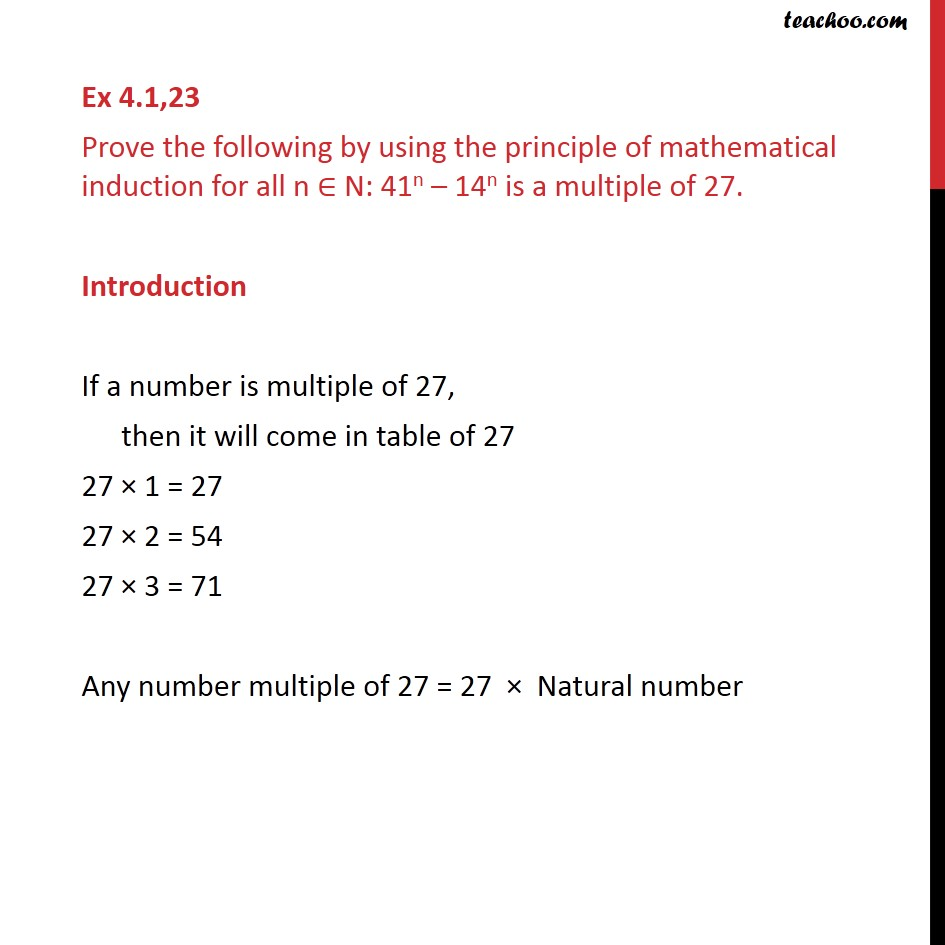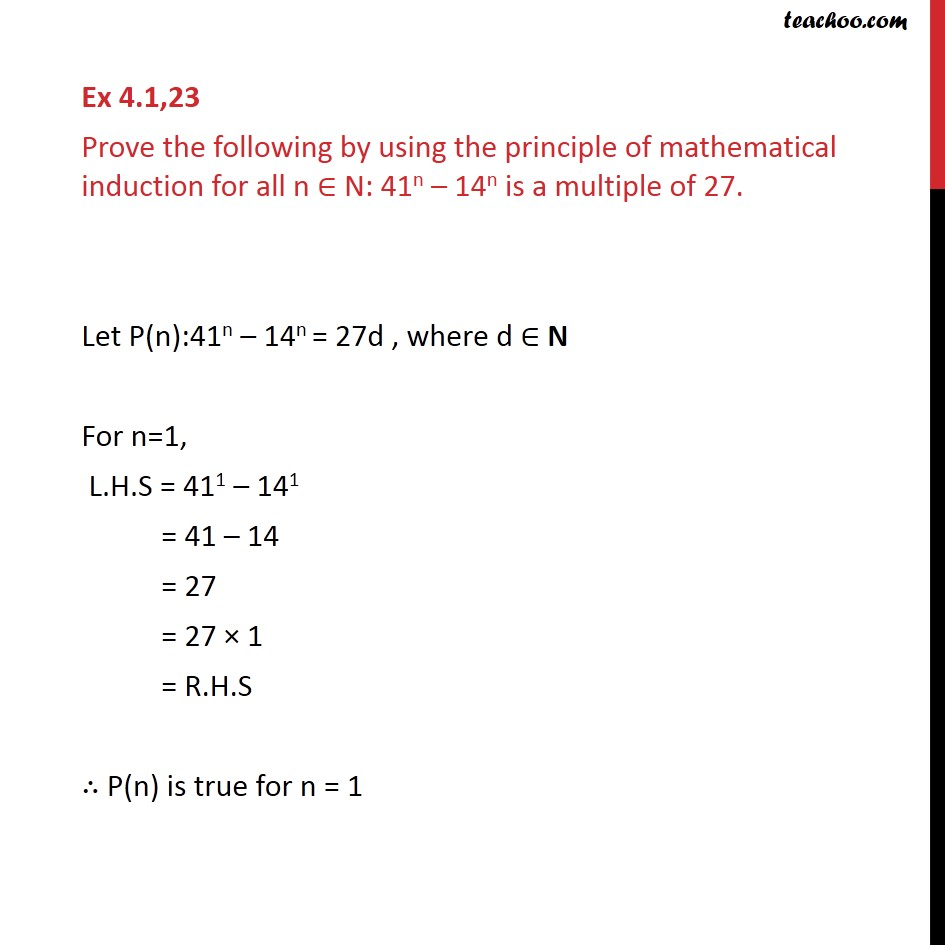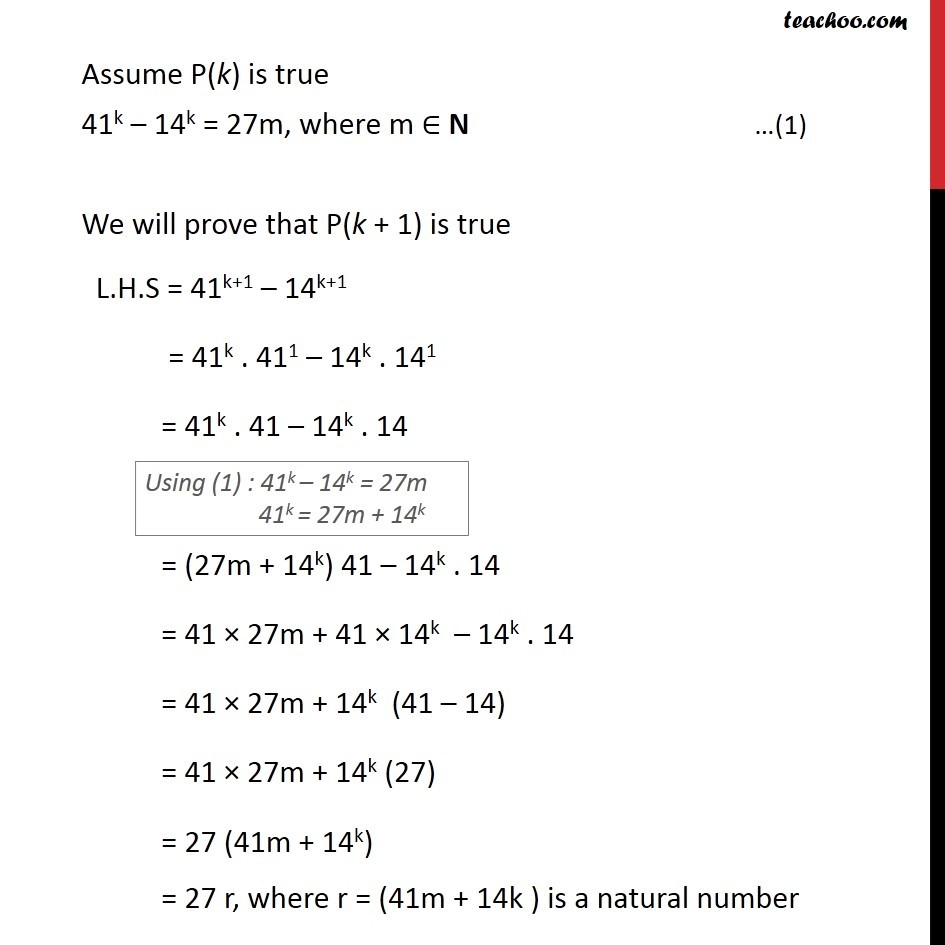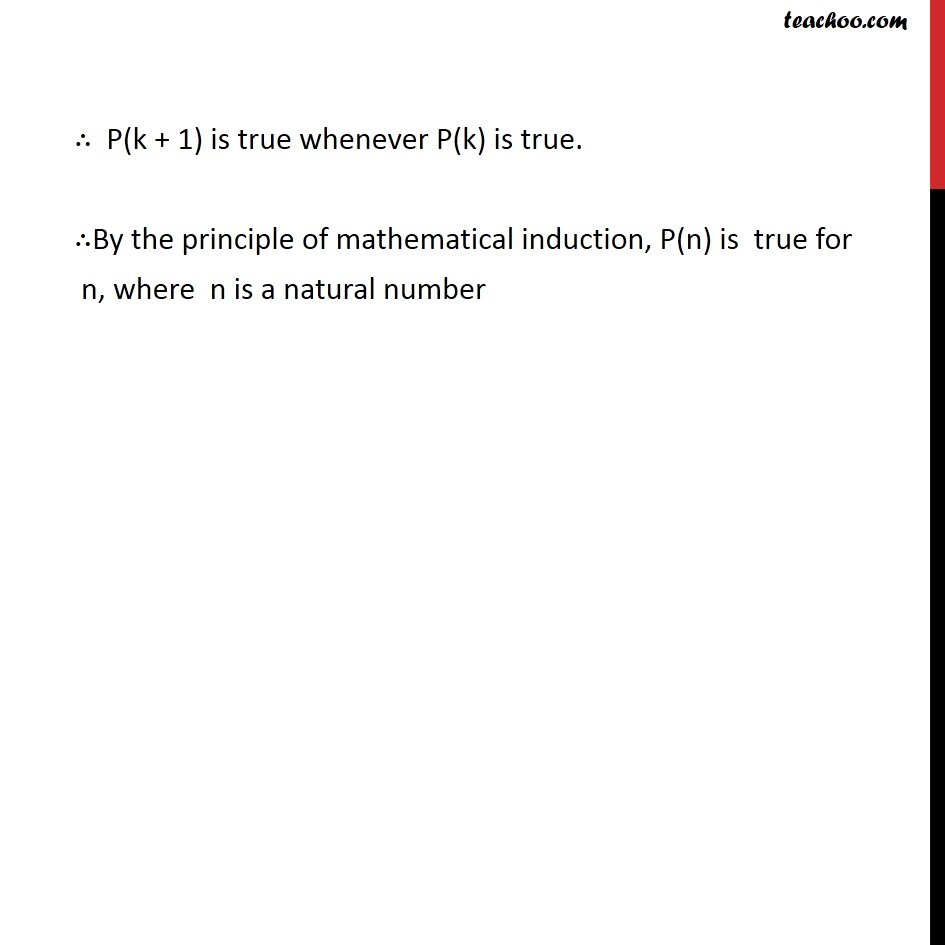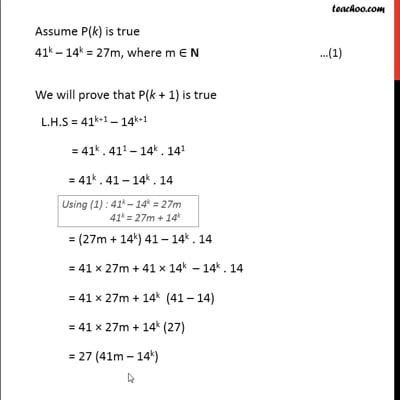This video is only available for Teachoo black users

Introducing your new favourite teacher - Teachoo Black, at only ₹83 per month

### Transcript

Ex 4.1,23 Prove the following by using the principle of mathematical induction for all n N: 41n 14n is a multiple of 27. Introduction If a number is multiple of 27, then it will come in table of 27 27 1 = 27 27 2 = 54 27 3 = 71 Any number multiple of 27 = 27 Natural number Ex 4.1,23 Prove the following by using the principle of mathematical induction for all n N: 41n 14n is a multiple of 27. Let P(n):41n 14n = 27d , where d N For n=1, L.H.S = 411 141 = 41 14 = 27 = 27 1 = R.H.S P(n) is true for n = 1 Assume P(k) is true 41k 14k = 27m, where m N We will prove that P(k + 1) is true L.H.S = 41k+1 14k+1 = 41k . 411 14k . 141 = 41k . 41 14k . 14 = (27m + 14k) 41 14k . 14 = 41 27m + 41 14k 14k . 14 = 41 27m + 14k (41 14) = 41 27m + 14k (27) = 27 (41m 14k) = 27 r, where r = (41m 14k ) is a natural number P(k + 1) is true whenever P(k) is true. By the principle of mathematical induction, P(n) is true for n, where n is a natural number